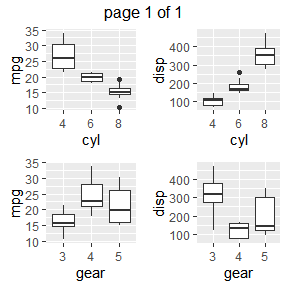Visualization

Introduction

In this document, we will introduce you to functions for generating different types of plots.

Continuous Data

The following functions will create plots for all or subset of continuous variables in the data set.

Histograms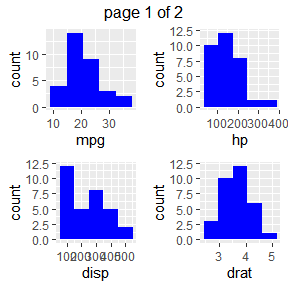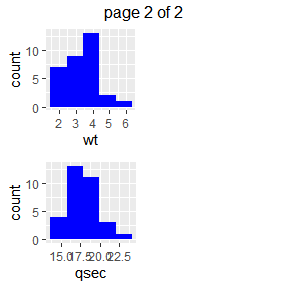Density Plots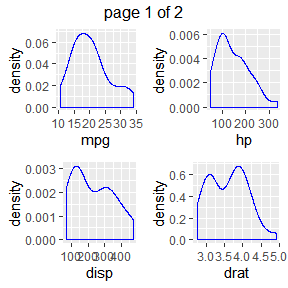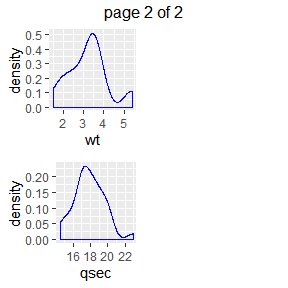Box Plots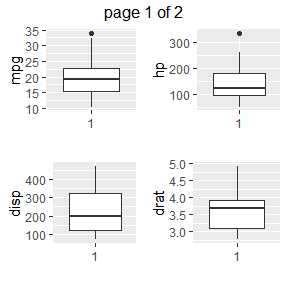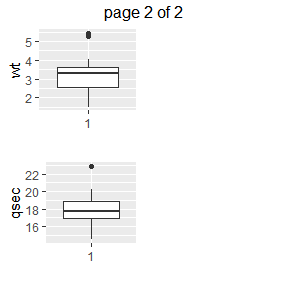Scatter Plots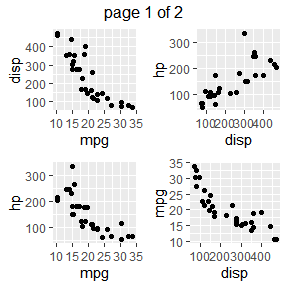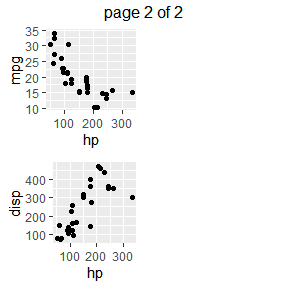Categorical Data

The following functions will create plots for all or subset of categorical variables in the data set.

Bar Plot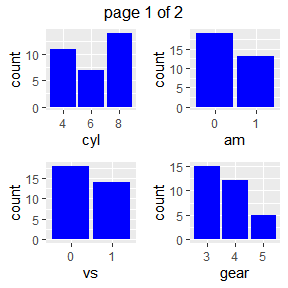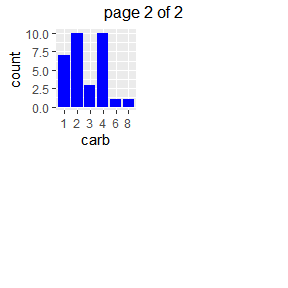Stacked Bar Plot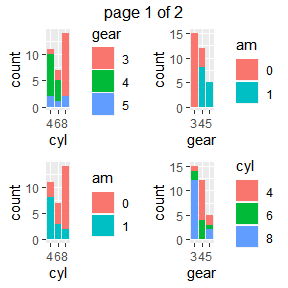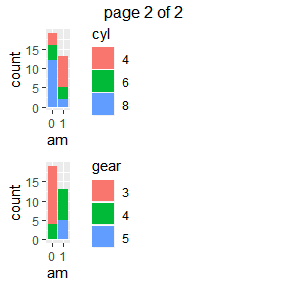Grouped Bar Plot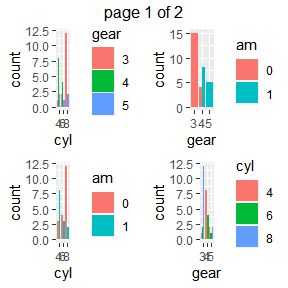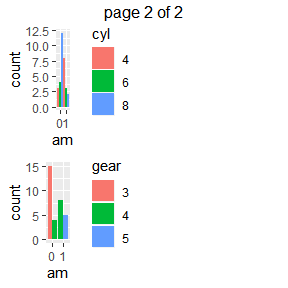Grouped Box Plots